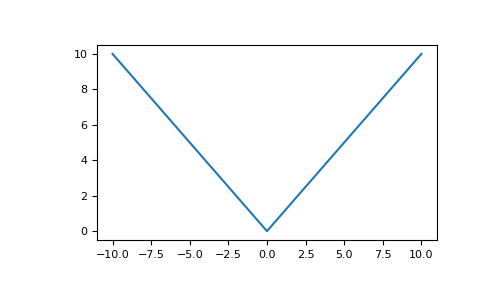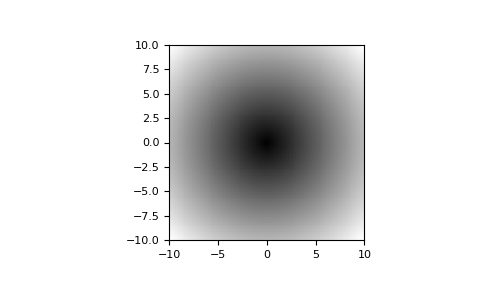numpy.square

numpy.fabs

# numpy.absolute¶

numpy.absolute(x, /, out=None, *, where=True, casting='same_kind', order='K', dtype=None, subok=True[, signature, extobj]) = <ufunc 'absolute'>

Calculate the absolute value element-wise.

np.abs is a shorthand for this function.

Parameters: x : array_like Input array. out : ndarray, None, or tuple of ndarray and None, optional A location into which the result is stored. If provided, it must have a shape that the inputs broadcast to. If not provided or None, a freshly-allocated array is returned. A tuple (possible only as a keyword argument) must have length equal to the number of outputs. where : array_like, optional Values of True indicate to calculate the ufunc at that position, values of False indicate to leave the value in the output alone. **kwargs For other keyword-only arguments, see the ufunc docs. absolute : ndarray An ndarray containing the absolute value of each element in x. For complex input, a + ib, the absolute value is. This is a scalar if x is a scalar.

Examples

>>> x = np.array([-1.2, 1.2])
>>> np.absolute(x)
array([ 1.2,  1.2])
>>> np.absolute(1.2 + 1j)
1.5620499351813308


Plot the function over [-10, 10]:

>>> import matplotlib.pyplot as plt

>>> x = np.linspace(start=-10, stop=10, num=101)
>>> plt.plot(x, np.absolute(x))
>>> plt.show()Plot the function over the complex plane:

>>> xx = x + 1j * x[:, np.newaxis]
>>> plt.imshow(np.abs(xx), extent=[-10, 10, -10, 10], cmap='gray')
>>> plt.show()